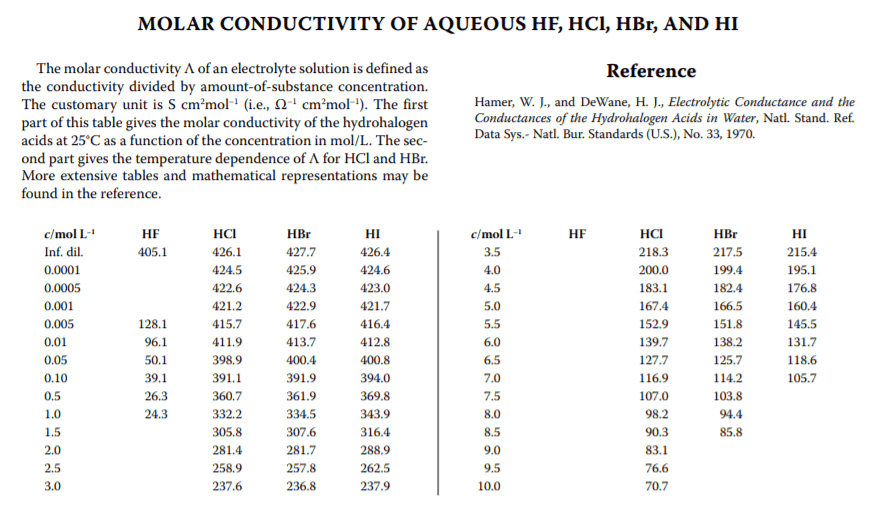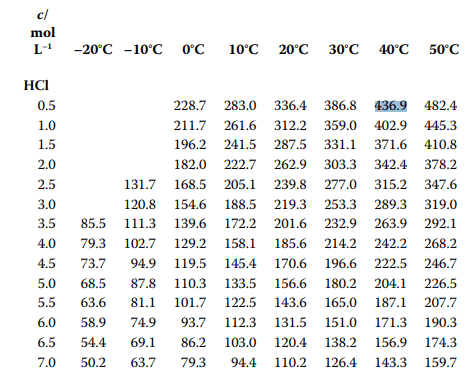# Calculate the HCl concentration in H2O from the conductivity

• gpsimms

#### gpsimms

TL;DR Summary
Mostly in the title: I am creating HCl in a tube reactor and crashing it out into a water trap. I am measuring pH and conductivity in the trap, and I want to say how many moles of HCl I have created.
Hi y'all,

I think this is my first time in the chemistry section of this forum, so thank you in advance for your patience with what is (I'm pretty sure) an elementary question. I've googled around for this a bit, but my last acid chemistry study was in 2002 or so, so the reading I am doing is not helping me.

I have an experiment which is creating HCl. I want to measure how much HCl my reactor is making, so the products are bubbled through a chilled water bath. I have real-time measurements of both pH and conductivity.

Once I get to low pH, the pH measurement is not sensitive enough to detect changes, due to log scale, so I also need to calculate HCl concentration from conductivity.

Conductivity is measured on my Hach instrument in mS/cm. Googling around led me to this table (below) for molar conductivity, but I'm a bit confused about the units. Below, it says molar conductivity is S*cm^2/mol. So does that mean that I need to take my measurement and multiply by volume of solution? And then divide by the number of moles HCl? But how do I do that, when that is my unknown?

Another question: I am starting with 2 L of H2O, and finishing with a pH around 1.6. Am I adding enough HCl that I need to consider a volume change to the water when determining concentration?How many digits to the right of the decimal does your pH meter display? If only one, then:

pH 1.5 is 10-1.5 = 3.2 X 10-2 mol/L
pH 1.6 is 10-1.6 = 2.5 X 10-2 mol/L
pH 1.7 is 10-1.7 = 2.0 X 10-2 mol/L

You need to monitor more closely than this? (~5-6 millimolar resolution or roughly +/- 3 mmol)
An extra digit resolution on the pH meter gets you an order of magnitude more resolution on concentration.

pH will determine concentration. To convert that to total #moles you will need to measure final volume. Evaporation effects may be something that affects final volume as well, so a final measurement of volume will solve for all unknowns.

Last edited:
To be honest, I wouldn't trust second decimal digit of the measured pH too much. Plus, converting pH to concentration without taking ionic strength of solution into account means your calculated concentration is substantially off (error in a 10% range for 0.03 M solutions).

pH of 1.6 means concentration of about 1g/L. That means volume changes are on the order of 0.1%, IMHO they are completely negligible (especially compared to the error mentioned above).

This unit thing is rather trivial, wikipedia page on conductivity of solutions gives all the necessary information, there is a simple dependence between concentration, specific conductivity and molar conductivity. Molar conductivity is actually not constant and changes with the concentration (again, ionic strength plus several other effects result in nonlinearity).

To be honest, I wouldn't trust second decimal digit of the measured pH too much. Plus, converting pH to concentration without taking ionic strength of solution into account means your calculated concentration is substantially off (error in a 10% range for 0.03 M solutions).
How far off would a conductivity measurement be?

Thank you all for the help so far. I have tried to take my data and calculate #moles HCl by using both pH and conductance.

The answers disagree significantly, so I think I must be doing something wrong, and would like to check.

For one case, my final pH is 1.66, and my final conductance is 21.47 mS/cm.

I started with 2 L of H2O, but did not measure the final volume (it sounds like maybe this should be done in future experiments...).

Using pH:

$$10^{-1.66}=0.02188$$
$$0.02188 \frac{moles}{L} \cdot 2L = 0.04376$$

So 0.04 moles HCl.

Now, using conductivity my final measurement is 21.47 mS/cm. Also, the water's temperature was being held constant at ##15^{o}##C. Now, it looks like on the table I posted that there is also a pretty strong temperature dependence on conductivity (see below):Now, our concentration is off this chart. So using the first chart, and the trend in this chart I *guessed* that the conductivity might be 330000 mS*cm^2/mol @ 0.1 moles @ 15 degrees C. *If you are wondering why I used 0.1 moles instead of 0.01 moles like the pH calculation suggests I should, I'll come back to this...*

Which gives this calculation:
(measured conductance [mS/cm]) ##\div## (molar conductivity [mS cm^2/mol]) = (concentration [mol/cm^3])

so...

$$21.47 \frac{ms}{cm} \times \frac{1 mol}{330000 mS \cdot cm^{2}} = 6.51\cdot 10^{-5} \frac{mol}{cm^{3}}$$

And we have approx 2000 cm^3, so ##6.51\cdot10^{-5}\cdot 2000=.13## moles. *This is why I used 0.1 moles to calc the molar conductivity.* Which disagrees with my pH calculation by a factor of 3. Which of the two measurements/calculations would you mistrust? Or did I screw something up in either of the calculations?

Finally, if anyone knows of a better table or a polynomial for HCl conductance, so I am not guessing the number there, please let me know.

Thanks!

Last edited:
Now look at the activity of HCl at pH 1.66 and you will see that the activity coefficient is ~0.9. Dividing the concentration by 0.9 would get you much closer to the concentration. To get spot on, you should make a series of standard HCl solutions (0.015, 0.020, 0.025 mol/L) and measure pH and conductance at 15C. Be sure to use the proper conductance electrode for this range and use the pH 1.68 standard buffer to calibrate the pH electrode/meter.

•BillTre